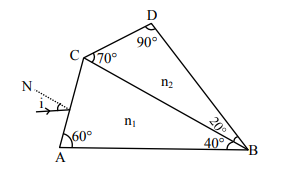# A prism of refractive index n1 and another prism of refractive index n2 are stuck together (as shown in the figure).`
Question:

A prism of refractive index $n_{1}$ and another prism of refractive index $\mathrm{n}_{2}$ are stuck together (as shown in the figure). $\mathrm{n}_{1}$ and $\mathrm{n}_{2}$ depend on $\lambda$, the wavelength of light, according to the relation

$\mathrm{n}_{1}=1.2+\frac{10.8 \times 10^{-14}}{\lambda^{2}}$ and $\mathrm{n}_{2}=1.45+\frac{1.8 \times 10^{-14}}{\lambda^{2}}$

The wavelength for which rays incident at any angle on the interface BC pass through without bending at that interface will be _________ nm.Solution:

For no bending, $\mathrm{n}_{1}=\mathrm{n}_{2}$

$1.2+\frac{10.8 \times 10^{-14}}{\lambda^{2}}=1.45+\frac{1.8 \times 10^{-4}}{\lambda^{2}}$

On solving,

$9 \times 10^{-14}=25 \lambda^{2}$

$\lambda=6 \times 10^{-7}$

$\lambda=600 \mathrm{~nm}$